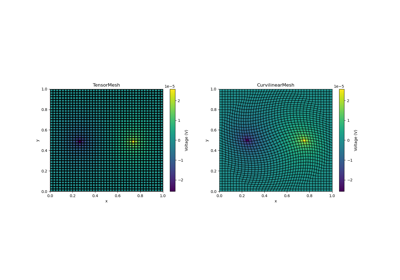# discretize.utils.closest_points_index#

discretize.utils.closest_points_index(mesh, pts, grid_loc='CC', **kwargs)[source]#

Find the indicies for the nearest grid location for a set of points.

Parameters:
mesh`discretize.base.BaseMesh`

An instance of discretize.base.BaseMesh

pts(`n`, `dim`) `numpy.ndarray`

Points to query.

grid_loc{‘CC’, ‘N’, ‘Fx’, ‘Fy’, ‘Fz’, ‘Ex’, ‘Ex’, ‘Ey’, ‘Ez’}

Specifies the grid on which points are being moved to.

Returns:
(`n` ) `numpy.ndarray` `of` `int`

Vector of length n containing the indicies for the closest respective cell center, node, face or edge.

Examples

Here we define a set of random (x, y) locations and find the closest cell centers and nodes on a mesh.

```>>> from discretize import TensorMesh
>>> from discretize.utils import closest_points_index
>>> import numpy as np
>>> import matplotlib.pyplot as plt
>>> h = 2*np.ones(5)
>>> mesh = TensorMesh([h, h], x0='00')
```

Define some random locations, grid cell centers and grid nodes,

```>>> xy_random = np.random.uniform(0, 10, size=(4,2))
>>> xy_centers = mesh.cell_centers
>>> xy_nodes = mesh.nodes
```

Find indicies of closest cell centers and nodes,

```>>> ind_centers = closest_points_index(mesh, xy_random, 'CC')
>>> ind_nodes = closest_points_index(mesh, xy_random, 'N')
```

Plot closest cell centers and nodes

```>>> fig = plt.figure(figsize=(5, 5))
>>> ax = fig.add_axes([0.1, 0.1, 0.8, 0.8])
>>> mesh.plot_grid(ax=ax)
>>> ax.scatter(xy_random[:, 0], xy_random[:, 1], 50, 'k')
>>> ax.scatter(xy_centers[ind_centers, 0], xy_centers[ind_centers, 1], 50, 'r')
>>> ax.scatter(xy_nodes[ind_nodes, 0], xy_nodes[ind_nodes, 1], 50, 'b')
>>> plt.show()
```

## Galleries and Tutorials using `discretize.utils.closest_points_index`#Basic Forward 2D DC Resistivity

Basic Forward 2D DC Resistivity